## Lemoine Point

The point of concurrenceof the Symmedian Lines, sometimes also called the Symmedian Point and Grebe Point.

Letbe the Centroid of a Triangle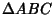,,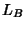, andthe Angle Bisectors of Angles,,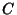, and,, andthe reflections of,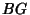, andabout,, and. Thenis the point of concurrence of the lines,, and. It is the perspectivity center of a Triangle and its Tangential Triangle.

In Areal Coordinates (actual Trilinear Coordinates), the Lemoine point is the point for whichis a minimum. A centeris the Centroid of its own Pedal Triangle Iff it is the Lemoine point.

The Lemoine point lies on the Brocard Axis, and its distances from the Lemoine pointto the sides of the Triangle are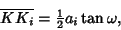whereis the Brocard Angle. A Brocard Line, Median, and Lemoine point are concurrent, with,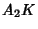, and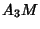meeting at a point. Similarly,,, andmeet at a point which is the Isogonal Conjugate of the first (Johnson 1929, pp. 268-269). The line joining the Midpoint of any side to the midpoint of the Altitude on that side passes through the Lemoine point. The Lemoine pointis the Steiner Point of the first Brocard Triangle.

See also Angle Bisector, Brocard Angle, Brocard Axis, Brocard Diameter, Centroid (Triangle), Cosymmedian Triangles, Grebe Point, Isogonal Conjugate, Lemoine Circle, Lemoine Line, Line at Infinity, Mittenpunkt, Pedal Triangle, Steiner Points, Symmedian Line, Tangential Triangle

References

Gallatly, W. The Modern Geometry of the Triangle, 2nd ed. London: Hodgson, p. 86, 1913.

Honsberger, R. Episodes in Nineteenth and Twentieth Century Euclidean Geometry. Washington, DC: Math. Assoc. Amer., 1995.

Johnson, R. A. Modern Geometry: An Elementary Treatise on the Geometry of the Triangle and the Circle. Boston, MA: Houghton Mifflin, pp. 217, 268-269, and 271-272, 1929.

Kimberling, C. Central Points and Central Lines in the Plane of a Triangle.'' Math. Mag. 67, 163-187, 1994.

Kimberling, C. Symmedian Point.'' http://cedar.evansville.edu/~ck6/tcenters/class/sympt.html.

Mackay, J. S. Early History of the Symmedian Point.'' Proc. Edinburgh Math. Soc. 11, 92-103, 1892-1893.